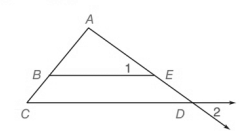Chapter 5.CR, Problem 18CRElementary Geometry For College St...

7th Edition
Alexander + 2 others
ISBN: 9781337614085

Solutions

Chapter
SectionElementary Geometry For College St...

7th Edition
Alexander + 2 others
ISBN: 9781337614085
Textbook Problem

Given:   ∠ 1 ≅ ∠ 2 .Prove: A B A C = B E C DTo determine

To prove:

ABAC=BECD

Explanation

Given:

12 and

Calculation:

By definition of alternate angles “a pair of angles on the inner side of each of two lines but on opposite sides of the traversal”.

Here, the alternate interior angles are CDE and 2

That is

Then by the theorem,

“If a line is parallel to one side of a triangle and intersects the other two sides, then it divides the sides proportionally”

In the above given figure, ΔACD with BE

Still sussing out bartleby?

Check out a sample textbook solution.

See a sample solution

The Solution to Your Study Problems

Bartleby provides explanations to thousands of textbook problems written by our experts, many with advanced degrees!

Get Started

Find more solutions based on key concepts